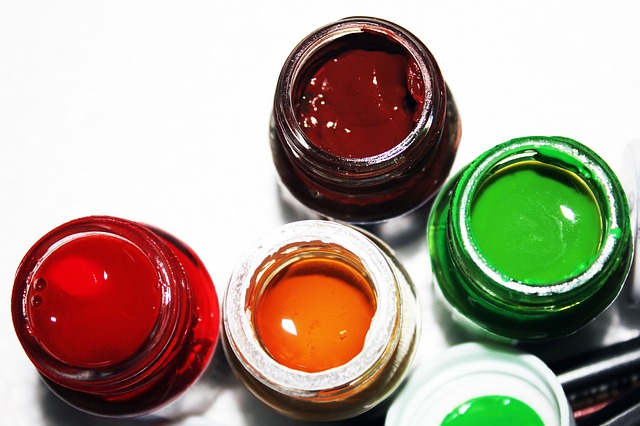# Double Number Lines# Introduction to Double Number Lines

A company will enlarge 5-inch-by-7-inch photographs to any size you want.

You can use a double number line to help you see some of the possible photograph sizes.

• To make a double number line, you start with two number lines and line them up at 0.
• You know that the ratio of the width of the original photograph to its length is 5:7, so you would make one tick mark on the top line at 5 and another tick mark on the second line at 7, making sure that the 5 and 7 are lined up.
• Now you can add other numbers to the number lines.
• What are some of the possible photograph sizes you can order?
• What is another pair of numbers that would line up on this double number line?
• What does each lined-up pair of numbers represent? (Think of the question from the problem.)## Opening

Use double number lines to solve ratio problems.

# Baseball Fields

Each of the measurements of a high school's softball field is in a 2:3 ratio to the corresponding measurements of the state’s major league baseball team field.

• Using the double number line tool, make a double number line that represents this ratio. Then use your double number line to answer the following questions.
• The distance from home plate to first base on the high school field is 20 yards. What is the distance in yards from home plate to first base on the major league field?
• The distance from home plate to the left field fence on the major league field is 120 yards. What is the distance in yards from home plate to the left field fence on the high school field?

INTERACTIVE: Double Number Line Tool

• What does each lined-up pair of numbers on your double number line represent?
• Where do you see 20 yards on the line representing the high school field?
• Where do you see 120 yards on the line representing the major league field?

# Recipes

Ratios are important in cooking. No matter what amount you make, you need to use the right ratio of ingredients in a recipe for the dish to taste the same every time.

Suppose that the recipe for an oil-and-vinegar dressing requires 6 tablespoons of salad oil and 2 tablespoons of vinegar.

• Create a double number line that shows this relationship. Use the Double Number Line Tool if you find it to be helpful.
• Use your double number line to determine how many tablespoons of salad oil you would need if you had 1 tablespoon of vinegar.
• How many tablespoons of vinegar would you need if you had 15 tablespoons of salad oil?

INTERACTIVE: Double Number Line Tool

What number on the line representing the vinegar should you line up with the 6 on the line representing the salad oil?

# Prepare a Presentation

Provide your solutions to each question. Write your solutions using complete sentences.

Explain how you used double number lines to solve each of the problems.

Compare the way you used the double number lines with the way you could use a tape diagram to solve these problems.

# Challenge Problem

In the problem about the baseball fields, the ratio of the high school field dimensions to the major league field dimensions is 2:3.

Suppose a local little league team wants to build a baseball field with measurements in a 1:3 ratio to those of the major league field.

• How would your double number line change?
• What would the ratio be between the little league field and the high school field?# Ways of Thinking: Make Connections

Take notes about how your classmates used the double number line to solve the problems and how they compared the double number line to the tape diagram.

• Can you explain the meaning of the pairs of numbers that line up on the double number line?
• How did you make the double number line for the salad dressing recipe?
• Do you think it’s easier to use a double number line or a tape diagram to solve ratio problems?

# Summary of the Math: Different Ways to Represent Ratios

• You can use both tape diagrams and double number lines to show ratios.
• This tape diagram shows that the ratio of pints of red paint to pints of blue paint in a paint mixture is 4:7.
• Thus, one way to produce a mixture with the correct ratio is to mix 4 pints of red paint with 7 pints of blue paint.
• You can use a double number line if you want to show many different ways to mix the paint and still get the same ratio of red to blue (and thus the same final paint color).
• This double number line shows that you can mix 4 pints of red paint with 7 pints of blue paint, or 8 pints of red paint with 14 pints of blue paint, or 12 pints of red paint with 21 pints of blue paint, and so on.
• You will still get a paint mixture that has a 4:7 ratio of red paint to blue paint.

Can you:

• Explain how to use tape diagrams to represent ratios?
• Explain how to use double number lines to represent ratios?

# Reflection

Write a reflection about the ideas discussed in class today. Use the sentence starters below if you find them to be helpful.

I think a tape diagram is similar to a double number line because …

I think a tape diagram is different from a double number line because …

Can you:

• Explain how to use tape diagrams to represent ratios?
• Explain how to use double number lines to represent ratios?